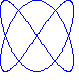#Lissajous choice

A Lissajous curve is a parametric curve in dimension 2, described by a pair of functions

x = cos(nt) , y = sin(mt+a) .

These curves occur often in the study of electronics. They form a good example of parametric curves.

This online exercise can either plot a Lissajous curve and ask you to recognize its equations among a list to choose from, or give a pair of equations and ask you to recognize the plotted Lissajous curve among a list to choose from.

Configuration of the exercise:
• Style of the exercise: .
• Type de variations : .
• A session will be composed of questions. (With a score assigned at the end of each session.)

Other exercises on: Lissajous   parametric curves